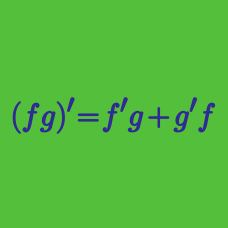Calculus

# Differentiation Rules Problem Solving

Consider a function $f(x)$ satisfying $f(x)=f(4x-2)$ for all real values $x$. If $f(x)$ is differentiable for all $x$ and $f'(4)=40,$ what is the value of $f'(54)?$

Given $\frac{d}{dx} \ln \frac{x+2}{\sqrt{x-7}}=\frac{f(x)}{2(x+2)(x-7)},$ what is $f(x)?$

The polynomial $P(x)$ satisfies the following identity: $P \left( P(x) + x \right) = 11 \left( P(x) + x \right)^2 - 4 \left( P(x) + x \right) + 5 .$ What is the value of $P'(6)?$

Given $g(x)=x \sin^{-1} \left(\frac{x}{24}\right)+\sqrt{576-x^2},$ what is the value of $g'(12)$?

Let $g(x)$ be the inverse function of a differentiable function $f(x).$ If $f(3)=7$ and $f'(3)=\frac{1}{2},$ what is the value of $\lim_{h \to 0} \frac{g(7+h)-g(7)}{h}?$

×